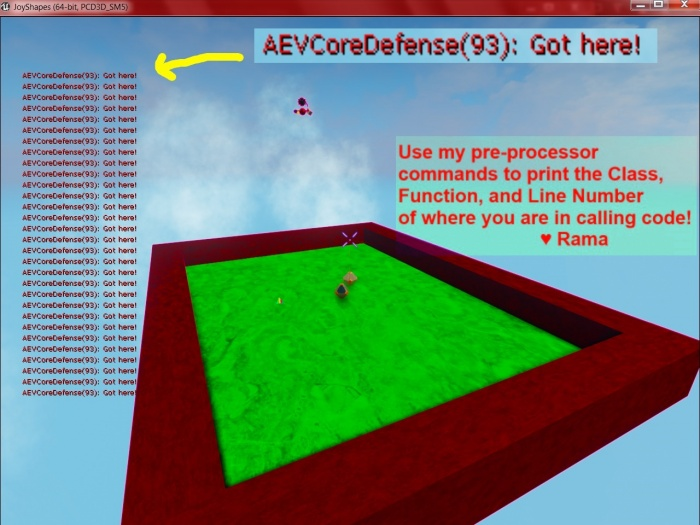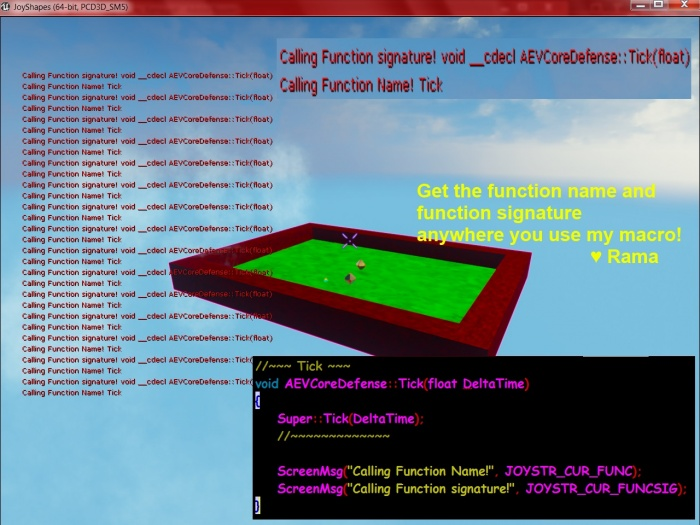# Logs, Printing Class Name, Function Name, Line Number of your Calling Code!

## Overview

Original Author: ( )

Dear Community,

In this wiki I am giving the code for how to independently print the Class, Function Name, and Line number wherever you are in the calling code that uses my pre-processor commands below!

You can even get a UE4 FString telling you the whole function signature of the function you are in where you use my code, including variable types!

## Print Screen Messages With Class/LineNumber

I also provide you with a pre-processor command that prints a message to the screen including the Class name and line number!

Here's a pic!After you get my .h file below, the code for the above picture is this!

```//~~~ Tick ~~~
void AEVCoreDefense::Tick(float DeltaTime)
{
Super::Tick(DeltaTime);
//~~~~~~~~~~~~~

VSCREENMSG("Got Here!");  //Class and line number get printed for you! ♥ Rama
}
```

## Print the name of the Calling Function, With Entire Signature!## My C++ Code For You

Here is the entire file you can #include in your code base!

I call it JoyCurrentClassFuncLine.h.

So you would then do this somewhere at the top of one of your core classes:

```// Joy Class Func Line
#include "JoyCurrentClassFuncLine.h"
```
```/*
Joy String
Current Class, File, and Line Number!
by Rama

PreProcessor commands to get
a. Class name
b. Function Name
c. Line number
d. Function Signature (including parameters)

Gives you a UE4 FString anywhere in your code that these macros are used!

Ex:
You can use JOYSTR_CUR_CLASS anywhere to get a UE4 FString back telling you
what the current class is where you called this macro!

Ex:
This macro prints the class and line along with the message of your choosing!
VSCREENMSG("Have fun today!");
<3  Rama
*/
#pragma once

//Current Class Name + Function Name where this is called!
#define JOYSTR_CUR_CLASS_FUNC (FString(__FUNCTION__))

//Current Class where this is called!
#define JOYSTR_CUR_CLASS (FString(__FUNCTION__).Left(FString(__FUNCTION__).Find(TEXT(":"))) )

//Current Function Name where this is called!
#define JOYSTR_CUR_FUNC (FString(__FUNCTION__).Right(FString(__FUNCTION__).Len() - FString(__FUNCTION__).Find(TEXT("::")) - 2 ))

//Current Line Number in the code where this is called!
#define JOYSTR_CUR_LINE  (FString::FromInt(__LINE__))

//Current Class and Line Number where this is called!
#define JOYSTR_CUR_CLASS_LINE (JOYSTR_CUR_CLASS + "(" + JOYSTR_CUR_LINE + ")")

//Current Function Signature where this is called!
#define JOYSTR_CUR_FUNCSIG (FString(__FUNCSIG__))

//Victory Screen Message
// 	Gives you the Class name and exact line number where you print a message to yourself!
#define VSCREENMSG(Param1) (GEngine->AddOnScreenDebugMessage(-1, 5.f, FColor::Red, *(JOYSTR_CUR_CLASS_LINE + ": " + Param1)) )

#define VSCREENMSG2(Param1,Param2) (GEngine->AddOnScreenDebugMessage(-1, 5.f, FColor::Red, *(JOYSTR_CUR_CLASS_LINE + ": " + Param1 + " " + Param2)) )

#define VSCREENMSGF(Param1,Param2) (GEngine->AddOnScreenDebugMessage(-1, 5.f, FColor::Red, *(JOYSTR_CUR_CLASS_LINE + ": " + Param1 + " " + FString::SanitizeFloat(Param2))) )

//UE LOG!
#define V_LOG(LogCat, Param1) 		UE_LOG(LogCat,Warning,TEXT("%s: %s"), *JOYSTR_CUR_CLASS_LINE, *FString(Param1))

#define V_LOG2(LogCat, Param1,Param2) 	UE_LOG(LogCat,Warning,TEXT("%s: %s %s"), *JOYSTR_CUR_CLASS_LINE, *FString(Param1),*FString(Param2))

#define V_LOGF(LogCat, Param1,Param2) 	UE_LOG(LogCat,Warning,TEXT("%s: %s %f"), *JOYSTR_CUR_CLASS_LINE, *FString(Param1),Param2)

#define V_LOGM(LogCat, FormatString , ...) UE_LOG(LogCat,Warning,TEXT("%s: %s"), 	*JOYSTR_CUR_CLASS_LINE, *FString::Printf(TEXT(FormatString), ##__VA_ARGS__ ) )
```

## V_LOG

Each of the V_LOG Macros takes as the first parameter the log category that you want to use!

```#define V_LOG(LogCat, Param1)   UE_LOG(LogCat,Warning,TEXT("%s: %s"), *JOYSTR_CUR_CLASS_LINE, *FString(Param1))
```

### V_LOGM

V_LOGM is special in that you can have an arbitrary number of vars that you output, and of any type, similar to standard UE_LOG functionality!

Example usage:

```int32 Health = 100;
float ArmorPct = 52.33;
FVector Location(33,12,1);

V_LOGM(Joy, "Health: %d, ArmorPct: %f, Loc: %s",  Health, ArmorPct, *Location.ToString());
```